# Tamil Nadu Board Samacheer Kalvi solutions for Class 4th Mathematics Term 2 Answers Guide chapter 2 - Numbers [Latest edition]

#### Chapters## Chapter 2: Numbers

Exercise 2.1Exercise 2.2Exercise 2.3Exercise 2.4Exercise 2.5Exercise 2.6Exercise 2.7
Exercise 2.1 [Pages 12 - 13]

### Tamil Nadu Board Samacheer Kalvi solutions for Class 4th Mathematics Term 2 Answers Guide Chapter 2 NumbersExercise 2.1 [Pages 12 - 13]

Exercise 2.1 | Q 1. (i) | Page 12

Multiply the following numbers by using lattice method.

52 × 49

Exercise 2.1 | Q 1. (ii) | Page 12

Multiply the following numbers by using lattice method.

347 × 5

Exercise 2.1 | Q 1. (iii) | Page 12

Multiply the following numbers by using lattice method.

127 × 7

Exercise 2.1 | Q 1. (iv) | Page 12

Multiply the following numbers by using lattice method.

65 × 37

Exercise 2.1 | Q 1. (v) | Page 12

Multiply the following numbers by using lattice method.

789 × 4

Exercise 2.1 | Q 1. (vi) | Page 12

Multiply the following numbers by using lattice method.

37 × 29

Exercise 2.1 | Q 2. (i) | Page 12

Multiply the following numbers by using standard method.

72 × 29

Exercise 2.1 | Q 2. (ii) | Page 12

Multiply the following numbers by using standard method.

31 × 27

Exercise 2.1 | Q 2. (iii) | Page 12

Multiply the following numbers by using standard method.

93 × 42

Exercise 2.1 | Q 2. (iv) | Page 12

Multiply the following numbers by using standard method.

124 × 6

Exercise 2.1 | Q 2. (v) | Page 12

Multiply the following numbers by using standard method.

206 × 8

Exercise 2.1 | Q 2. (vi) | Page 12

Multiply the following numbers by using standard method.

479 × 3

Exercise 2.1 | Q 3. | Page 13

One box contains 25 apples. How many apples are there in 36 such boxes?

Exercise 2.1 | Q 3. i. | Page 13

There are 28 pages in a daily newspaper. If Ajay buys newspaper for 45 days, how many pages will it contain?

Exercise 2.1 | Q 3. ii. | Page 13

A truck carries 125 bags of rice. If each bag has 9 kg of rice, how much kg of rice will there be in the truck.

Exercise 2.1 | Q 3. iii. | Page 13

If the cost of one chair is ₹ 857. What will be the cost of 6 chairs?

Exercise 2.2 [Page 14]

### Tamil Nadu Board Samacheer Kalvi solutions for Class 4th Mathematics Term 2 Answers Guide Chapter 2 NumbersExercise 2.2 [Page 14]

Exercise 2.2 | Q 1 | Page 14

4 × 6 = square

Exercise 2.2 | Q 2 | Page 14

7 × 6 = square

Exercise 2.2 | Q 3 | Page 14

8 × 6 = square

Exercise 2.2 | Q 4 | Page 14

In a shirt there are 6 buttons, how many buttons will there be in 8 shirts?

Exercise 2.2 | Q 5 | Page 14

In a carton, there are 9 rows with 6 eggs in each row. How many eggs are there in all?

Exercise 2.2 | Q 6 | Page 14

Complete the 7th table: (using maths kit box). 7 Piece tan gram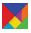7 × 1 = 7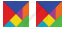7 × 2 = 14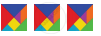7 × 3 = 21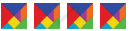7 × 4 = ______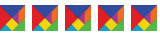7 × 5 = 35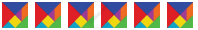_________________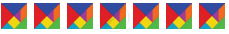7 × 7 = ________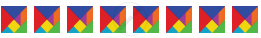7 × 8 = 56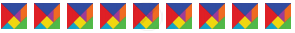_________________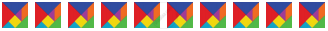7 × 10 = 70
Exercise 2.3 [Pages 14 - 15]

### Tamil Nadu Board Samacheer Kalvi solutions for Class 4th Mathematics Term 2 Answers Guide Chapter 2 NumbersExercise 2.3 [Pages 14 - 15]

Exercise 2.3 | Q 1 | Page 14

3 × 7 = square

Exercise 2.3 | Q 2 | Page 14

6 × 7 = square

Exercise 2.3 | Q 3 | Page 14

9 × 7 = square

Exercise 2.3 | Q 4 | Page 14

A box contains 7 pens. How many pens are there in 5 boxes?

Exercise 2.3 | Q 5 | Page 14

One week has 7 days. Calculate the number of days in 10 weeks?

Exercise 2.3 | Q 6 | Page 15

Complete the 8th table: Number of legs in spider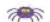8 × 1 = 8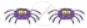8 × 2 = 16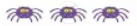8 × 3 = 24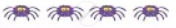8 × 4 = 32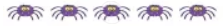8 × 5 = 40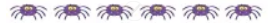______________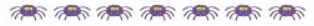8 × 7 = 56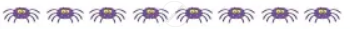8 × 8 = ______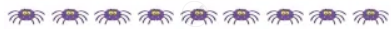8 × 9 = ______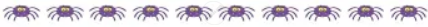8 × 10 = 80
Exercise 2.4 [Page 15]

### Tamil Nadu Board Samacheer Kalvi solutions for Class 4th Mathematics Term 2 Answers Guide Chapter 2 NumbersExercise 2.4 [Page 15]

Exercise 2.4 | Q 1 | Page 15

8 × 4 = square

Exercise 2.4 | Q 2 | Page 15

8 × 6 = square

Exercise 2.4 | Q 3 | Page 15

8 × 10 = square

Exercise 2.4 | Q 4 | Page 15

One packet contains 8 pencils, how many pencils are there in 9 such packets?

Exercise 2.4 | Q 5 | Page 15

The price of one ball is ₹ 10. Find the cost of 8 such balls?

Exercise 2.4 | Q 6 | Page 15
Complete the 9th table:

9 = 9 × 1 = 9
9 + 9 = 9 × 2 = 18
9 + 9 + 9 = 9 × 3 = 27
9 + 9 + 9 + 9 = 9 × 4 = 36
9 + 9 + 9 + 9 + 9 = 9 × 5 = 45
9 + 9 + 9 + 9 + 9 + 9 = 9 × 6 = ______________
9 + 9 + 9 + 9 + 9 + 9 + 9 = ______________
9 + 9 + 9 + 9 + 9 + 9 + 9 + 9 = 9 × 8 = ______________
9 + 9 + 9 + 9 + 9 + 9 + 9 + 9 + 9 = 9 × 9 = 81
9 + 9 + 9 + 9 + 9 + 9 + 9 + 9 + 9 + 9 = ______________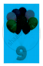9 × 1 = 99 × 2 = 18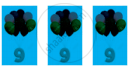9 × 3 = 27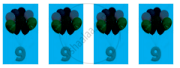9 × 4 = 36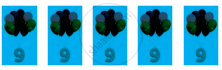9 × 5 = 45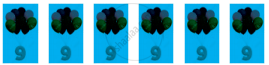9 × 6 = 54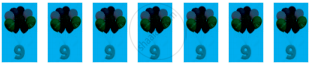9 × 7 = 63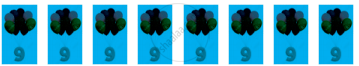______________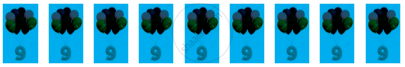9 × 9 = ______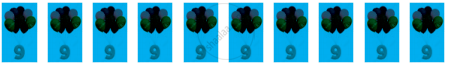9 × 10 = _____
Exercise 2.5 [Page 17]

### Tamil Nadu Board Samacheer Kalvi solutions for Class 4th Mathematics Term 2 Answers Guide Chapter 2 NumbersExercise 2.5 [Page 17]

Exercise 2.5 | Q 1 | Page 17

9 × 3 = square

Exercise 2.5 | Q 2 | Page 17

9 × 6 = square

Exercise 2.5 | Q 3 | Page 17

9 × 10 = square

Exercise 2.5 | Q 4 | Page 17

A sports team has 9 persons. How many persons will there be in 9 teams?

Exercise 2.5 | Q 5 | Page 17

Number of rods in a window is 9. Find the number of rods in 7 windows.

Exercise 2.5 | Q 6 | Page 17

Complete the 10th table:

From the tables 1 to 9 we will know the following:

Complete the following:

 10 × 1 = 10 10 × 1 = 10 10 × 2 = 20 10 × 2 = 20 10 × 3 = 30 10 × 3 = 30 10 × 4 = 40 10 × 4 = 40 10 × 5 = 50 _____________ 10 × 6 = 60 _____________ 10 × 7 = 70 _____________ 10 × 8 = 80 _____________ 10 × 9 = 90 10 × 9 = 90 10 × 10 = 100 _____________
Exercise 2.6 [Page 18]

### Tamil Nadu Board Samacheer Kalvi solutions for Class 4th Mathematics Term 2 Answers Guide Chapter 2 NumbersExercise 2.6 [Page 18]

#### Fill in the boxes.

Exercise 2.6 | Q 1 | Page 18

Multiply by 10, 100, 1000

Fill in the boxes.

1. 10 × 7 = 70
2. 100 × 16 = square
3. 1000 × 9 = 9000
4. 10 × 696 = square
5. 100 × 96 = 9600
6. 1000 × 6 = square
Exercise 2.7 [Page 19]

### Tamil Nadu Board Samacheer Kalvi solutions for Class 4th Mathematics Term 2 Answers Guide Chapter 2 NumbersExercise 2.7 [Page 19]

Exercise 2.7 | Q 1 | Page 19

Write the number as the product of two numbers.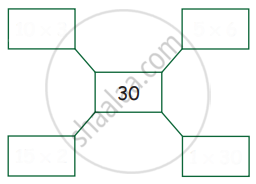Exercise 2.7 | Q 2 | Page 19

Write the number as the product of two numbers.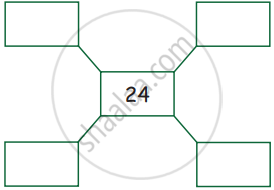Exercise 2.7 | Q 3 | Page 19

Write the number as the product of two numbers.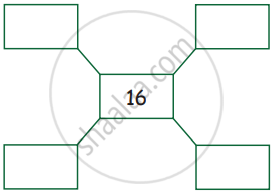Exercise 2.7 | Q 4 | Page 19

Write the number as the product of two numbers.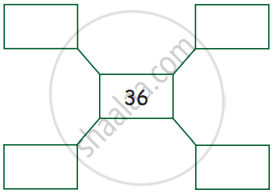## Chapter 2: Numbers

Exercise 2.1Exercise 2.2Exercise 2.3Exercise 2.4Exercise 2.5Exercise 2.6Exercise 2.7## Tamil Nadu Board Samacheer Kalvi solutions for Class 4th Mathematics Term 2 Answers Guide chapter 2 - Numbers

Tamil Nadu Board Samacheer Kalvi solutions for Class 4th Mathematics Term 2 Answers Guide chapter 2 (Numbers) include all questions with solution and detail explanation. This will clear students doubts about any question and improve application skills while preparing for board exams. The detailed, step-by-step solutions will help you understand the concepts better and clear your confusions, if any. Shaalaa.com has the Tamil Nadu Board of Secondary Education Class 4th Mathematics Term 2 Answers Guide solutions in a manner that help students grasp basic concepts better and faster.

Further, we at Shaalaa.com provide such solutions so that students can prepare for written exams. Tamil Nadu Board Samacheer Kalvi textbook solutions can be a core help for self-study and acts as a perfect self-help guidance for students.

Concepts covered in Class 4th Mathematics Term 2 Answers Guide chapter 2 Numbers are Multiplication by Lattice Algorithm ( up to 2 Digit Number by 2 Digit Number and 3 Digit Number by Single Digit Number).

Using Tamil Nadu Board Samacheer Kalvi Class 4th solutions Numbers exercise by students are an easy way to prepare for the exams, as they involve solutions arranged chapter-wise also page wise. The questions involved in Tamil Nadu Board Samacheer Kalvi Solutions are important questions that can be asked in the final exam. Maximum students of Tamil Nadu Board of Secondary Education Class 4th prefer Tamil Nadu Board Samacheer Kalvi Textbook Solutions to score more in exam.

Get the free view of chapter 2 Numbers Class 4th extra questions for Class 4th Mathematics Term 2 Answers Guide and can use Shaalaa.com to keep it handy for your exam preparation